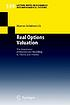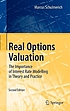# Classify

## Summary

Title:
Real options valuation : the importance of interest rate modelling in theory and practice
Author:
Formats:

Editions:
47
Total Holdings:
1314
OCLC Work Id:
793615749
http://classify.oclc.org/classify2/ClassifyDemo?owi=793615749
Most Frequent 332.63 786 Web Dewey
Edition: 23 332 24 Web Dewey
Most Frequent HG6042 790 ClassWeb## Editions

Displaying 1 to 25 of 47
Title and AuthorLanguageFormatHoldings TagClass NumberReal options valuation : the importance of interest rate modelling in theory and practiceby Schulmerich, Marcuseng 349082332.63

(DDC 22)

050HG6042Real options valuation : the importance of interest rate modelling in theory and practiceby Schulmerich, Marcuseng 331082658.152

(DDC 22)

050HG6042Real options valuation : the importance of interest rate modelling in theory and practiceby Schulmerich, Marcuseng 286050HG4028.C4
082332.63

(DDC 22)Real options valuation : the importance of interest rate modelling in theory and practiceby Schulmerich, Marcuseng 105082332.63

(DDC 21)

050HG4028.C4Real options valuation : the importance of interest rate modelling in theory and practiceby Schulmerich, Marcuseng 32050HG6042
082658.15

(DDC 22)Real options valuation : the importance of interest rate modelling in theory and practiceby Schulmerich, Marcuseng 30050HG6042
082332.6Real Options Valuation : the Importance of Interest Rate Modelling in Theory and Practiceeng 25082332Real options valuation : the importance of interest rate modelling in theory and practiceby Schulmerich, Marcuseng 23050HG6042Real Options Valuation : the Importance of Interest Rate Modelling in Theory and Practiceby Schulmerich, Marcuseng 13082332

(DDC 23)Real options valuation : the importance of interest rate modelling in theory and practiceby Schulmerich, Marcuseng 12082330Real Options Valuationby Schulmerich, Marcuseng 12082332.63Real Options Valuation : the Importance of Interest Rate Modelling in Theory and Practiceeng 11082332

(DDC 23)Real options valuation : the importance of interest rate modelling in theory and practiceby Schulmerich, Marcuseng 10050HG4028.C4
082332.63

(DDC 22)Real options valuation : the importance of interest rate modelling in theory and practiceby Schulmerich, Marcuseng 6082332.63228

(DDC 21)Real options valuation : the importance of interest rate modelling in theory and practiceby Schulmerich, Marcuseng 4050HG6042Real options valuation : the importance of interest rate modelling in theory and practiceby Schulmerich, Marcuseng 4050HG4028.C4Real Options Valuation : the Importance of Interest Rate Modelling in Theory and Practiceby Schulmerich, Marcuseng 4050HG6042Real Options Valuation : the Importance of Interest Rate Modelling in Theory and Practiceby Schulmerich, Marcuseng 4050HB172.5
082332.63Real Options Valuation : the Importance of Interest Rate Modelling in Theory and Practiceby Schulmerich, Marcuseng 4050HB172.5
082332.63Real Options Valuation : the Importance of Interest Rate Modelling in Theory and Practiceby Schulmerich, Marcuseng 4050HG6042Real Options Valuation : The Importance of Interest Rate Modelling in Theory and Practiceby Schulmerich, Marcuseng 3050HB172.5
082332.63Real options valuation : the importance of interest rate modelling in theory and practiceby Schulmerich, Marcuseng 3050HG4028.C4
082332.63

(DDC 21)Real options valuation : the importance of interest rate modelling in theory and practiceby Schulmerich, Marcuseng 3050HG4028.C4Real options valuation : the importance of interest rate modelling in theory and practiceby Schulmerich, Marcuseng 3050HG6042
082658.152

(DDC 22)Real Options Valuation : The Importance of Interest Rate Modelling in Theory and Practiceby Schulmerich, Marcuseng 3
Displaying 1 to 25 of 47
.